7月QCon广州站2022，关注Web 3.0、数据架构选型、数字化转型等热门话题，点击了解 了解详情

# TensorFlow 工程实战（三）：结合知识图谱实现电影推荐系统

• 2019 年 8 月 14 日
• 本文字数：7653 字

阅读完需：约 25 分钟## 实例描述

### 一、准备数据集

• item_index2entity_id.txt：电影的 ID 与序号。具体内容如图 1 所示，第 1 列是电影 ID，第 2 列是序号。

• kg.txt：电影的知识图谱。图 2 中显示了知识图谱的 SPO 三元组（Subject-Predicate-Object），第 1 列是电影 ID，第 2 列是关系，第 3 列是目标实体。

• ratings.dat：用户的评分数据集。具体内容如图 3 所示，列与列之间用“::”符号进行分割，第 1 列是用户 ID，第 2 列是电影 ID，第 3 列是电影评分，第 4 列是评分时间（可以忽略）。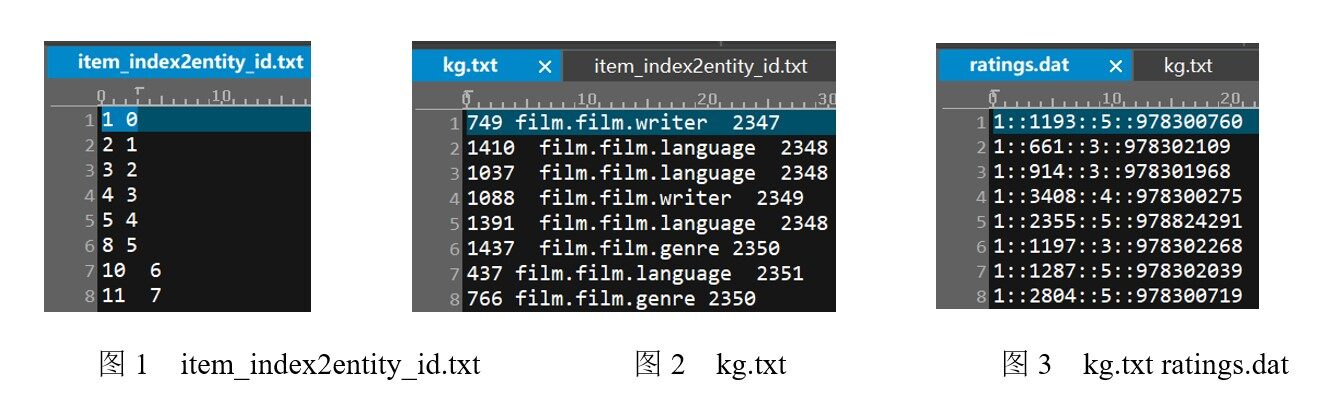### 二、预处理数据

• kg_final.txt：转化后的知识图谱文件。将文件 kg.txt 中的字符串类型数据转成序列索引类型数据，如图 4 所示。

• ratings_final.txt：转化后的用户评分数据集。第 1 列将 ratings.dat 中的用户 ID 变成序列索引。第 2 列没有变化。第 3 列将 ratings.dat 中的评分按照阈值 5 进行转化，如果评分大于等于 5，则标注为 1，表明用户对该电影感兴趣。否则标注为 0，表明用户对该电影不感兴趣。具体内容如图 5 所示。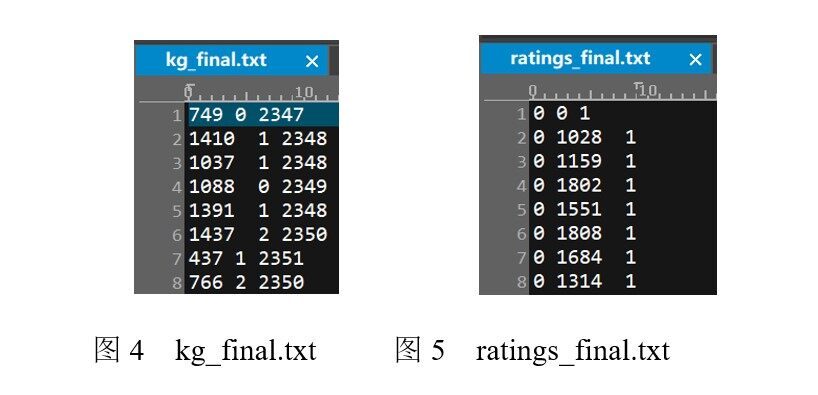### 三、搭建 MKR 模型

MKR 模型由 3 个子模型组成，完整结构如图 6 所示。具体描述如下。

• 推荐算法模型：如图 6 的左侧部分所示，将用户和电影作为输入，模型的预测结果为用户对该电影的喜好分数，数值为 0～1。

• 交叉压缩单元模型：如图 6 的中间部分，在低层将左右两个模型桥接起来。将电影评分数据集中的电影向量与知识图谱中的电影向量特征融合起来，再分别放回各自的模型中，进行监督训练。

• 知识图谱词嵌入（Knowledge Graph Embedding，KGE）模型：如图 6 的右侧部分，将知识图谱三元组中的前 2 个（电影 ID 和关系实体）作为输入，预测出第 3 个（目标实体）。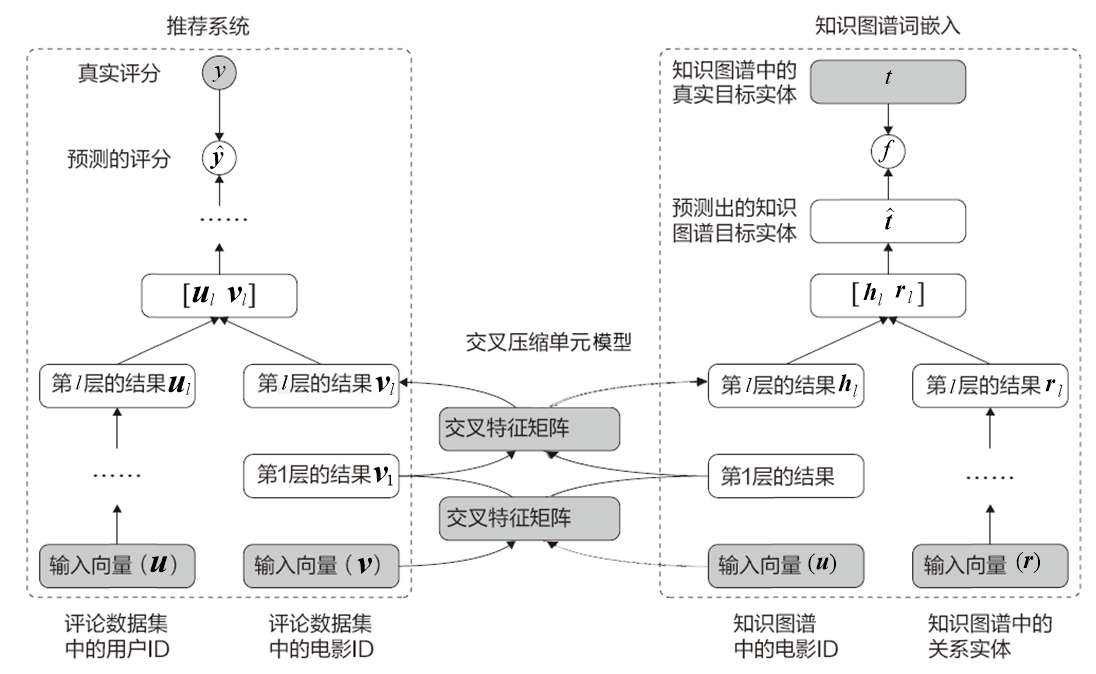#### 1. 交叉压缩单元模型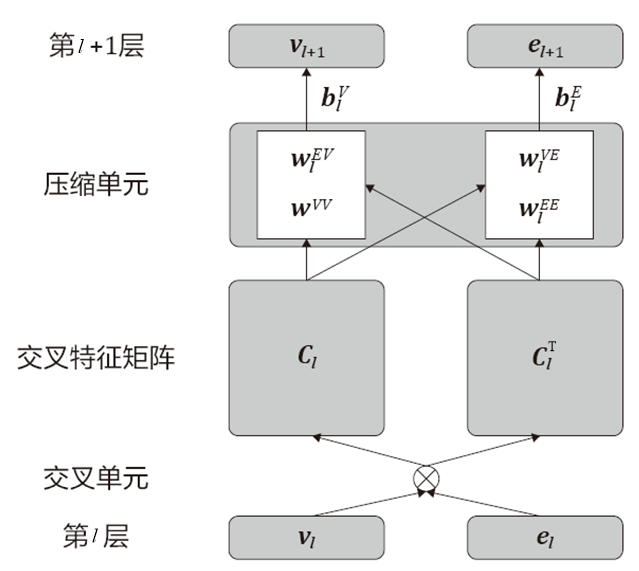（1）将 v_l 与 e_l 进行矩阵相乘得到 c_l。

（2）将 c_l 复制一份，并进行转置得到 c_l^T。实现特征交叉融合。

（3）将 c_l 经过权重矩阵 w_l^vv 进行线性变化（c_l 与 w_l^vv 矩阵相乘）。

（4）将 c_l^T 经过权重矩阵 w_l^ev 进行线性变化。

（5）将（3）与（4）的结果相加，再与偏置参数 b_l^v 相加，得到 v_(l+1)。v_(l+1)将用于推荐算法模型的后续计算。

（6）按照第（3）、（4）、（5）步的做法，同理可以得到 e_(l+1)。e_(l+1)将用于知识图谱词嵌入模型的后续计算。

import numpy as npimport tensorflow as tffrom sklearn.metrics import roc_auc_scorefrom tensorflow.python.layers import baseclass CrossCompressUnit(base.Layer):                      #定义交叉压缩单元模型类    def __init__(self, dim, name=None):       super(CrossCompressUnit, self).__init__(name)       self.dim = dim       self.f_vv = tf.layers.Dense(1, use_bias = False)  #构建权重矩阵       self.f_ev = tf.layers.Dense(1, use_bias = False)       self.f_ve = tf.layers.Dense(1, use_bias = False)       self.f_ee = tf.layers.Dense(1, use_bias = False)       self.bias_v = self.add_weight(name='bias_v',     #构建偏置权重                                           shape=dim,                                            initializer=tf.zeros_initializer())         self.bias_e = self.add_weight(name='bias_e',                                            shape=dim,                                           initializer=tf.zeros_initializer())    def _call(self, inputs):        v, e = inputs          #v和e的形状为[batch_size, dim]        v = tf.expand_dims(v, dim=2)  #v的形状为 [batch_size, dim, 1]        e = tf.expand_dims(e, dim=1)  #e的形状为 [batch_size, 1, dim]        c_matrix = tf.matmul(v, e)#c_matrix的形状为 [batch_size, dim, dim]        c_matrix_transpose = tf.transpose(c_matrix, perm=[0, 2, 1])        #c_matrix的形状为[batch_size * dim, dim]        c_matrix = tf.reshape(c_matrix, [-1, self.dim])        c_matrix_transpose = tf.reshape(c_matrix_transpose, [-1, self.dim])        #v_output的形状为[batch_size, dim]        v_output = tf.reshape(                           self.f_vv(c_matrix) + self.f_ev(c_matrix_transpose),                          [-1, self.dim]                                 ) + self.bias_v        e_output = tf.reshape(                          self.f_ve(c_matrix) + self.f_ee(c_matrix_transpose),                          [-1, self.dim]                                  ) + self.bias_e    #返回结果    return v_output, e_output

#### 2. 将交叉压缩单元模型集成到 MKR 框架中

• 低层：将所有的输入映射成词嵌入向量，将需要融合的向量（图 6 中的 v 和 h）输入交叉压缩单元，不需要融合的向量（图 6 中的 u 和 r）进行同步的全连接层处理。

• 高层：推荐算法模型和知识图谱词嵌入模型分别将低层的传上来的特征连接在一起，通过全连接层回归到各自的目标结果。

class MKR(object):    def __init__(self, args, n_users, n_items, n_entities, n_relations):        self._parse_args(n_users, n_items, n_entities, n_relations)        self._build_inputs()        self._build_low_layers(args)   #构建低层模型        self._build_high_layers(args)   #构建高层模型        self._build_loss(args)        self._build_train(args)    def _parse_args(self, n_users, n_items, n_entities, n_relations):        self.n_user = n_users        self.n_item = n_items        self.n_entity = n_entities        self.n_relation = n_relations        #收集训练参数，用于计算l2损失        self.vars_rs = []        self.vars_kge = []    def _build_inputs(self):        self.user_indices=tf.placeholder(tf.int32, [None], 'userInd')        self.item_indices=tf.placeholder(tf.int32, [None],'itemInd')        self.labels = tf.placeholder(tf.float32, [None], 'labels')        self.head_indices =tf.placeholder(tf.int32, [None],'headInd')        self.tail_indices =tf.placeholder(tf.int32, [None], 'tail_indices')        self.relation_indices=tf.placeholder(tf.int32, [None], 'relInd')    def _build_model(self, args):         self._build_low_layers(args)        self._build_high_layers(args)    def _build_low_layers(self, args):        #生成词嵌入向量        self.user_emb_matrix = tf.get_variable('user_emb_matrix',                                                       [self.n_user, args.dim])        self.item_emb_matrix = tf.get_variable('item_emb_matrix',                                                       [self.n_item, args.dim])        self.entity_emb_matrix = tf.get_variable('entity_emb_matrix',                                                       [self.n_entity, args.dim])        self.relation_emb_matrix = tf.get_variable('relation_emb_matrix',                                                      [self.n_relation, args.dim])        #获取指定输入对应的词嵌入向量，形状为[batch_size, dim]        self.user_embeddings = tf.nn.embedding_lookup(                                      self.user_emb_matrix, self.user_indices)        self.item_embeddings = tf.nn.embedding_lookup(                                      self.item_emb_matrix, self.item_indices)        self.head_embeddings = tf.nn.embedding_lookup(                                     self.entity_emb_matrix, self.head_indices)        self.relation_embeddings = tf.nn.embedding_lookup(                              self.relation_emb_matrix, self.relation_indices)        self.tail_embeddings = tf.nn.embedding_lookup(                                   self.entity_emb_matrix, self.tail_indices)        for _ in range(args.L):#按指定参数构建多层MKR结构            #定义全连接层            user_mlp = tf.layers.Dense(args.dim, activation=tf.nn.relu)            tail_mlp = tf.layers.Dense(args.dim, activation=tf.nn.relu)            cc_unit = CrossCompressUnit(args.dim)#定义CrossCompress单元            #实现MKR结构的正向处理            self.user_embeddings = user_mlp(self.user_embeddings)            self.tail_embeddings = tail_mlp(self.tail_embeddings)            self.item_embeddings, self.head_embeddings = cc_unit(                               [self.item_embeddings, self.head_embeddings])            #收集训练参数            self.vars_rs.extend(user_mlp.variables)            self.vars_kge.extend(tail_mlp.variables)             self.vars_rs.extend(cc_unit.variables)            self.vars_kge.extend(cc_unit.variables)                            def _build_high_layers(self, args):        #推荐算法模型        use_inner_product = True     #指定相似度分数计算的方式        if use_inner_product:        #内积方式            #self.scores的形状为[batch_size]            self.scores = tf.reduce_sum(self.user_embeddings * self.item_embeddings, axis=1)        else:            #self.user_item_concat的形状为[batch_size, dim * 2]            self.user_item_concat = tf.concat(                       [self.user_embeddings, self.item_embeddings], axis=1)            for _ in range(args.H - 1):                rs_mlp = tf.layers.Dense(args.dim * 2, activation=tf.nn.relu)                #self.user_item_concat的形状为[batch_size, dim * 2]                self.user_item_concat = rs_mlp(self.user_item_concat)                self.vars_rs.extend(rs_mlp.variables)            #定义全连接层            rs_pred_mlp = tf.layers.Dense(1, activation=tf.nn.relu)            #self.scores的形状为[batch_size]            self.scores = tf.squeeze(rs_pred_mlp(self.user_item_concat))            self.vars_rs.extend(rs_pred_mlp.variables)  #收集参数        self.scores_normalized = tf.nn.sigmoid(self.scores)        #知识图谱词嵌入模型        self.head_relation_concat = tf.concat(  #形状为[batch_size, dim * 2]                  [self.head_embeddings, self.relation_embeddings], axis=1)        for _ in range(args.H - 1):            kge_mlp = tf.layers.Dense(args.dim * 2, activation=tf.nn.relu)            #self.head_relation_concat的形状为[batch_size, dim* 2]            self.head_relation_concat = kge_mlp(self.head_relation_concat)            self.vars_kge.extend(kge_mlp.variables)        kge_pred_mlp = tf.layers.Dense(args.dim, activation=tf.nn.relu)        #self.tail_pred的形状为[batch_size, args.dim]        self.tail_pred = kge_pred_mlp(self.head_relation_concat)        self.vars_kge.extend(kge_pred_mlp.variables)        self.tail_pred = tf.nn.sigmoid(self.tail_pred)        self.scores_kge = tf.nn.sigmoid(tf.reduce_sum(self.tail_embeddings * self.tail_pred, axis=1))        self.rmse = tf.reduce_mean(            tf.sqrt(tf.reduce_sum(tf.square(self.tail_embeddings - self.tail_pred), axis=1) / args.dim))

• 使用内积的方式，计算用户向量和电影向量的相似度。有关相似度的更多知识，可以参考 8.1.10 小节的注意力机制。

• 将用户向量和电影向量连接起来，再通过全连接层处理计算出用户对电影的喜好分值。

（1）将电影向量和知识图谱中的关系向量连接起来。

（2）将第（1）步的结果通过全连接层处理，得到知识图谱三元组中的目标实体向量。

（3）将生成的目标实体向量与真实的目标实体向量矩阵相乘，得到相似度分值。

（4）对第（3）步的结果进行激活函数 sigmoid 计算，将值域映射到 0～1 中。

#### 3. 实现 MKR 框架的反向结构

MKR 框架的反向结构主要是 loss 值的计算，其 loss 值一共分为 3 部分：推荐算法模型模型的 loss 值、知识图谱词嵌入模型的 loss 值和参数权重的正则项。具体实现的代码如下。

    def _build_loss(self, args):        #计算推荐算法模型的loss值        self.base_loss_rs = tf.reduce_mean(            tf.nn.sigmoid_cross_entropy_with_logits(labels=self.labels, logits=self.scores))        self.l2_loss_rs = tf.nn.l2_loss(self.user_embeddings) + tf.nn.l2_loss (self.item_embeddings)        for var in self.vars_rs:            self.l2_loss_rs += tf.nn.l2_loss(var)        self.loss_rs = self.base_loss_rs + self.l2_loss_rs * args.l2_weight        #计算知识图谱词嵌入模型的loss值        self.base_loss_kge = -self.scores_kge        self.l2_loss_kge = tf.nn.l2_loss(self.head_embeddings) + tf.nn.l2_loss (self.tail_embeddings)        for var in self.vars_kge:   #计算L2正则            self.l2_loss_kge += tf.nn.l2_loss(var)        self.loss_kge = self.base_loss_kge + self.l2_loss_kge * args.l2_weight    def _build_train(self, args):   #定义优化器        self.optimizer_rs = tf.train.AdamOptimizer(args.lr_rs).minimize(self.loss_rs)        self.optimizer_kge = tf.train.AdamOptimizer(args.lr_kge). minimize(self. loss_kge)    def train_rs(self, sess, feed_dict):    #训练推荐算法模型        return sess.run([self.optimizer_rs, self.loss_rs], feed_dict)    def train_kge(self, sess, feed_dict):     #训练知识图谱词嵌入模型        return sess.run([self.optimizer_kge, self.rmse], feed_dict)    def eval(self, sess, feed_dict):      #评估模型        labels, scores = sess.run([self.labels, self.scores_normalized], feed_dict)        auc = roc_auc_score(y_true=labels, y_score=scores)        predictions = [1 if i >= 0.5 else 0 for i in scores]        acc = np.mean(np.equal(predictions, labels))        return auc, acc    def get_scores(self, sess, feed_dict):        return sess.run([self.item_indices, self.scores_normalized], feed_dict)

### 四、训练模型并输出结果

……

epoch 9 train auc: 0.9540 acc: 0.8817 eval auc: 0.9158 acc: 0.8407 test auc: 0.9155 acc: 0.8399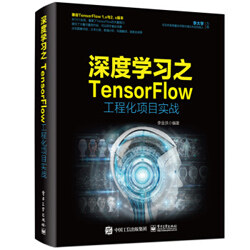TensorFlow 工程实战（三）：结合知识图谱实现电影推荐系统（本文）

2019 年 8 月 14 日 12:1010378

## 评论

• ###### 与其感慨模型难训练，不如试试 AutoNLP

你或许知道AutoML，但未必用过AutoNLP。

• ###### TensorFlow 工程实战（五）：构建 DeblurGAN 模型，将模糊相片变清晰

在拍照时，常常因为手抖或补光不足，导致拍出的照片很模糊。本文将介绍如何利用DeblurGAN模型将模糊的照片变清晰。

• ###### 苹果发布 Core ML 2

在WWDC大会上，苹果发布了Core ML 2：iOS设备的新版机器学习SDK。新版本Core ML 2将带来30％的推理速度提升。Core ML SDK的一个重要新功能是Create ML。开发者可以在Mac上使用Swift创建和训练自定义机器学习模型。

• ###### TensorFlow 工程实战（二）：用 tf.layers API 在动态图上识别手写数字

如何使用卷积网络在MNIST数据集上进行手写数字识别？

• ###### TensorFlow 工程实战（四）：使用带注意力机制的模型分析评论者是否满意

如何利用tf.keras接口搭建一个只带有注意力机制的模型，进而实现文本分类？

• ###### 18｜Wide&Deep：怎样让你的模型既有想象力又有记忆力？

Wide&Deep模型在业界有着巨大的影响力，只要掌握了它，我们就能抓住深度推荐模型这几年发展的一个主要方向。

2020 年 11 月 20 日

• ###### 港中文林达华团队提出计算机视觉新方向：电影情节分析

今天为大家带来的论文导读是来自商汤科技联合创始人、香港中文大学林达华教授团队提出的电影分析模型。这不仅是一个新的模型，更是计算机视觉领域一个全新的研究方向。在这篇文章中，作者提出了一个框架，综合了视觉模块和时间分析模块两部分功能。这两个模块从数据的不同集合中学习，视觉模块从预告片中学习，而时间分析模块则从电影中学习。这样既学习到了有效的视觉特征，又避免了大量计算，同时保持了整部电影的时序结构。

• ###### 离线评估：常用的推荐系统离线评估方法有哪些？

在推荐系统这个行业，所有人都在谈效果。那这个所谓的“效果”到底指的是什么呢？我们一般用什么方法来衡量这个“效果”呢？

2020 年 12 月 7 日

• ###### 07｜回归分析：怎样用模型预测用户的生命周期价值？

怎样用聚类分析预测用户的生命周期价值？

2021 年 9 月 13 日

• ###### 网易新闻推荐：深度学习排序系统及模型

本文介绍网易新闻推荐的深度学习排序系统和模型。

• ###### 基于深度学习的推荐模型之一：受限波兹曼机

这周，我们来讨论如何利用深度学习来提升推荐系统的精度。

2018 年 4 月 9 日

• ###### Python 数据挖掘与机器学习实战（四）：用 Python 实现多元线性回归

**编者按**：本文节选自方巍著《Python数据挖掘与机器学习实战》一书中的部分章节。

• ###### 如何模拟光照让 3D 场景更逼真？（下）

真实世界的反射模型，其实是由镜面反射和漫反射结合起来的。你想实现更真实的3D效果吗？那你必须要掌握Phong反射模型。

2020 年 8 月 17 日

• ###### 11｜深度学习（上）：用 CNN 带你认识深度学习

要构建出能够实现图像分类的CNN，我们通常需要把“卷积+池化”的组合重复搭建几次，形成深度卷积网络。

2021 年 9 月 22 日

• ###### 深入浅出 TensorFlow（六）TensorFlow 高层封装

为了让TensorFlow使用起来更加灵活，更加方便，可以使用一些高级封装。本文将介绍TensorFlow的四种主要封装：TensorFlow-Slim、tf.contrib.learn（之前也被称为skflow）、TFLearn、Keras；同时还将通过其中常用的三种方式在MNIST数据集上实现卷积神经网络。

• ###### 深入浅出 TensorFlow（二）：TensorFlow 解决 MNIST 问题入门

本文是整个系列的第二篇文章，将会简单介绍TensorFlow安装方法、TensorFlow基本概念、神经网络基本模型，并在MNIST数据集上使用TensorFlow实现一个简单的神经网络。

6月月更

6月月更

6月月更

#架构实战营

#### Linux知识库（3）- 命令行和包管理